Professional Pilot Training (includes ground studies) A forum for those on the steep path to that coveted professional licence. Whether studying for the written exams, training for the flight tests or building experience here's where you can hang out.

# ATPL theory questions

Join Date: Jan 2006
Location: Chester
Posts: 142
Likes: 0
Received 0 Likes on 0 Posts
ChriSat - thanks for your reply! I have no idea how I arrived at that either. I blame the calculator! I now see that I was working it out correctly, but I must have put in the wrong values or copied down the answer incorrectly.

pilotmike - thank you for your reply also. I see what you're saying, I meant to say 39 200kgs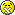Join Date: Dec 2007
Location: Meteorology Avenue
Age: 43
Posts: 186
Received 0 Likes on 0 Posts
PoF theory questions

Hi,

1.If an airplane glides at an angle of attack of 10�, how much altitude will it lose in 1 mile?
A:240 feet.
B: 480 feet.
C: 960 feet.

2.How much altitude will this airplane lose in 3 miles of gliding at an angle of attack of 8�?
A:440 feet.
B: 880 feet.
C: 1,320 feet.

The figure to use: 3_2 | Flickr - Photo Sharing!

Thanks!

Mxms

Last edited by maximus610; 13th Feb 2012 at 20:42.Join Date: Jan 2011
Location: England
Posts: 652
Received 0 Likes on 0 Posts
The questions are based n the equation:

Glide Range = Height x L/D ratio

Rearranging this gives

Height = Glide Range / L/D ratio.

The diagram shows that

L/D ratio at 10 degrees angle of attack = approximately 11.2

L/D ratio at 8 degress angle of attack = approximately 12.2

If we assume that the 1 mile and 3 mile in the question are nautical miles and assuming 1 nm = 1680 feet we get

For 10 degrees height = 6080 / 11.2 = 542 ft

For 8 degrees height = 6080 x 3 / 12.2 = 1495 feet

These answers are not very close to the options given

But if we assume that the 1 mile and 3 mile in the question are statute miles (5280 feet) we get

For 10 degrees height = 5280 / 11.2 = 471 ft

For 8 degrees height = 5280 x 3 / 12.2 = 1298 feet

These are closer to the options of 480 feet and 1320 feet.

These are very unusual questions and are clearly not from the JAR ATPL system. Where did they come from?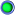Join Date: Aug 2008
Location: Lima, Peru
Posts: 10
Likes: 0
Received 0 Likes on 0 Posts
Hello everybody

They are from the Peruvian CPL question bank. I asked Max for help with the question because I am here doing a license conversion I don't have any books with me.

Great answer, thanks a lot!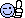CheersJoin Date: Oct 2000
Location: Bristol
Posts: 461
Likes: 0
Received 0 Likes on 0 Posts
Ola peruano

While you are here could you pse say how QNH is calculated at high altitude airfields like Cuzco and La Paz

DickJoin Date: Aug 2008
Location: Lima, Peru
Posts: 10
Likes: 0
Received 0 Likes on 0 Posts
ola gringoI guess it's calculated just like at any airfield in the world, that is adjusted to sea level.

SJoin Date: Oct 2000
Location: Bristol
Posts: 461
Likes: 0
Received 0 Likes on 0 Posts
Gracias S

I had an idea that for high altitude fields a different datum was used.

No soy gringo, soy ingles - as I have had to say many times in Ecuador

Cheers, DickJoin Date: Aug 2008
Location: Lima, Peru
Posts: 10
Likes: 0
Received 0 Likes on 0 Posts
Don't mention itI know you are ingles but I noticed that here they don't make the difference... it was meant as a joke, sorry about that.

Cheers
SJoin Date: Jul 2011
Location: UK
Posts: 53
Likes: 0
Received 0 Likes on 0 Posts
Altimetry Help

Good afternoon,

I think this is what it feels like to have mental block! I have just got 99 out of 100 altimetry questions correct, but I just can't work this one out for some reason. Maybe I'm staring too hard trying to find the right answer that I'm missing the obvious solution, but it's something I'd like to get clear in my head either way. You may ask why I'm so bothered when it's only one question out of 100 - well, because it's 'sods law' that it'll come up in the exam! This same question has been answered further up the thread, but it doesn't help solve the confusion in my case. Here goes:

"You plan a flight over a mountain range at a true altitude of 15000ft/AMSL. The air is on average 15C colder than than ISA, the pressure at sea level is 1003 hPa. What approximate altitude should the altimeter read (pressure setting 1013 hPa)?"

Now I'm quite good at remembering and re-arranging mathematical formulas as opposed to rules (just a personal preference), and so I use:

True Altitude = Altitude on QNH + [(ISA Deviation x 4) x (Pressure Altitude/1000)] and I've always got the answer to similar style questions spot on!

With the question above though, I can't get my head around which one of the missing components I'm actually looking for? We're given the True Altitude as 15,000ft, and I know that the ISA Deviation x 4 = -60, and so that means I either need to find the Altitude on the QNH or the pressure altitude, but I can't work out which.

Is anyone able to help explain using my formula? The answer by the way is supposedly 16,230', although I'm aware with these that the answers are quite often 50' out and you just have to pick the closest one.Join Date: Jan 2011
Location: England
Posts: 652
Received 0 Likes on 0 Posts

True Altitude = Altitude on QNH + [(ISA Deviation x 4) x (Pressure Altitude/1000)]

Gives

Alt on QNH = True Altitude - [(ISA Dev x 4) x (Pressure Alt/1000)]

Inserting the data provided gives

Alt on QNH = 15000 � ((-15 x 4 x 15) = 15900

But we are going to use 1013 which is 10 HPA higher than QNH.

Using 27 ft / Hpa this means that the 1013 level is 270 feet below msl

So adding 270 to 15900 gives an indicated altitude of 16170 feet.

At that altitude the estimated lapse rate of of 27 feet per Hpa is a bit too low. It looks as if the examiner has used 33 ft per HPa (30 HPa per 1000 ft) which is probably more realistic.Join Date: Jul 2011
Location: UK
Posts: 53
Likes: 0
Received 0 Likes on 0 Posts
That's brilliant Keith, I think I've pretty much got it now!

Am I right in thinking that you're effectively working out what the Altitude on the QNH would be (1003 set) and so therefore Pressure Altitude is the same as True Altitude in the initial calculation? And then you're taking in to account the variation between sea level QNH and 1013?Join Date: Jan 2011
Location: England
Posts: 652
Received 0 Likes on 0 Posts

It should be

TA = IA + (4 x IA/1000 x ISA Dev) + (27 x (QNH � Subscale))

Where
TA = true altitude,
IA = indicated altitude,
Subscale = altimeter subscale setting,
ISA Dev = ISA temperature deviation

Strictly speaking the term "Pressure Altitude" means the altimeter reading when 1013 is set on the subscale. So if we were to use Pressure Altitude instead of indicated altitude in the above equation we would be applying the temperature error from the 1013 level upwards.

My use of TA in ,y previous post was an error. Using the above equation we can solve the problem as follows.

TA = IA + (4 x IA/1000 x ISA Dev) + (27 x (QNH � Subscale))

ISA Deviation = -15
True Altitude = 15000 ft
QNH = 1003

TA � (27 x (QNH-Subscale setting) = IA + ( (4 x IA x ISA Dev)/1000 )

Inserting the data provided in the question gives

15000 � ( 27 x (1003 � 1013) = IA + (4 x IA x (�15) ) / 1000 )

15000 + 270 = IA � 0.06 IA

15270 = 0.94 IA

15270 / 0.94 = IA

16244 = IA

So Indicated Altitude = 16244 feet

This is not quite the correct answer of 16230, but it is pretty close to it.

Last edited by keith williams; 19th Feb 2012 at 17:25.Join Date: Jun 2010
Location: Stockholm
Posts: 25
Likes: 0
Received 0 Likes on 0 Posts
AC Electrics

Hey guys, I could use some input on this one.

If an alternator is run at below normal frequency, then:
A) Electric motors will stop.
B) Inductive devices will overheat.
C) Lights will become dim.
D) Lights will become brighter.

The correct(?) answer is B. I agree that if the frequency is below normal, that would mean that the inductive reactance is lower and thus the current will be higher, but conversely, a lower frequency would also mean that capacative reactance would be higher, thus giving a lower current, which should balance the lower inductive reactance, as there is no mention of one reactance being more dominant than the other, or any one kind of reactance at all for that matter, other than what might possibly be deducted when the answer alternatives are examined. Am I to assume that the other alternatives, being placed in a purely capacative reactant circuit couldn't occur in the case of decreased frequency?

-AndersJoin Date: Jan 2011
Location: England
Posts: 652
Received 0 Likes on 0 Posts
This is a case (just one of many) where the examiners have made some simplfiying assumptons, but have not stated what these assumptions were.

All they really want you to do is to note that reducing frequency reduces inductive reactance so for a given applied voltage the current through inductors will increase.

As you have said the situation is much more complicated in real circuits where different types of component are connected in various ways.

But if you look at the other options, the voltage regulator should prevent these effects unless the RPM is very much lower than standard.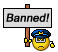Join Date: Apr 2009
Location: down south
Age: 76
Posts: 13,226
Likes: 0
Received 0 Likes on 0 Posts
Blimey Keith!

You must be bored.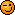Join Date: Nov 2008
Location: Land of Hope
Posts: 47
Likes: 0
Received 0 Likes on 0 Posts
What is a suction peak in terms of principles of flight.Join Date: Jun 2010
Location: Stockholm
Posts: 25
Likes: 0
Received 0 Likes on 0 Posts
Obviously I'm missing something, but hopefully some one here can help me out.

Question 1:
Given that the characteristics of a three engine turbojet aeroplane are as follows:
Trust = 50000 N per engine
g= 10 m/s�
Drag = 72569 N
Minimum gross gradient (2nd segment) = 2.7%
The maximum take-off mass under segment 2 conditions in the net take-off flight path conditions is:

a. 101596 kg
b. 286781 kg
c. 74064 kg
d. 209064 kg

Question 2:
The determination of the maximum mass on brake release, of a certified turbojet aeroplane with 5�, 15� and 25� flaps angles on take-off, leads to the following values, with wind:

Flap angle: 5� 15� 25�
Runway limitation: 66000 69500 71500
2nd segment slope limitation: 72200 69000 61800

Wind correction: Head wind: + 120 kg per kt OR tail wind: -360 kg per kt
Given that the tail wind component is equal to 5 kt, the maximum mass on brake release and corresponding flap angle will be:

a. 67700/15�
b. 69000/15�
c. 72200/5�
d. 69700/25�

Question 3:
On a segment of the take-off flight path an obstacle requires a minimum gradient of climb of 2.6% in order to provide an adequate margin of safe clearance. At a mass of 110000 kg the gradient of climb is 2.8%. For the same power and assuming that the angle of climb varies inversely with, at what maximum mass will the aeroplane be able to to achieve the minimum gradient?

a. 121310 kg
b. 106425 kg
c. 118455 kg
d. 102142 kg

It's too late for me to write in my calculations, and for the same reason, please excuse any typos.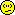-AndersJoin Date: Jan 2011
Location: England
Posts: 652
Received 0 Likes on 0 Posts
Question 1.
The first point to note in addressing this question is that calculation of the maximum take-off mass assumes that a single engine failure has occurred. This means that this three engine aircraft effectively has only two engines.

So total thrust available 2 x 50000N = 100000N.

For small angles of climb, the % climb = 100% x Sin angle of climb

This can be rearranged to give: Sin angle of climb = % climb /100

And Sin angle of climb = (Thrust � drag) / Weight

Combing the two equations above gives:% climb / 100 = (Thrust � Drag) / weight
This can be rearranged to give: Max weight = 100 x (Thrust-Drag) / % climb

Inserting the data provided in the question gives:

Max take-off weight = 100 x (100000 � 72569) / 2.7 = 1015962.963N

This can be converted into Kg by dividing by g = 10 m/s2 to give 101596.2963 Kg or approximately 101596 Kg.

Question 2.
To solve this type of problem it must first be noted that winds do not affect the climb limited take-off mass. The wind correction need therefore be applied only to the runway limit (field limited take-off mass).

The next stage of the solution is to calculate and apply the corrections. the question specifies a correction factor of -360 kg/kt tailwind and an actual tailwind of 5 kts. this gives a correction of -360 Kg/kt x 5kt = - 1800 Kg.

Adding this to the figures provided in the question gives:

Flap angle: 5� 15� 25 �
Runway limitation (kg): 64 200 67 700 69 700
2nd segment slope limitation: 72 200 69 000 61 800

Finally select the flap setting for which the lower of the two limits is greatest. This is 15degree flap which gives a limit of 67 700 Kg.

Question 3.
The maximum climb gradient that an aircraft can achieve is inversely proportional to its mass. This can be stated in the form of an equation:

%climb at new mass x new mass = % climb at old mass x old mass

This can be rearranged to give:

New mass = % climb at old mass x old mass
% climb at new mass

Inserting the data provided in the question gives:

New mass = 2.8% x 110000 Kg = 118461.54 Kg
2.6%Join Date: Jun 2010
Location: Stockholm
Posts: 25
Likes: 0
Received 0 Likes on 0 Posts
Yes, but of course. Where I went wrong in question 1 was that I was counting with the thrust from all 3 engines. And in question 2, I was making the wind correction to the climb limit also. Thanks for clearing that up for me. However, when it comes to question 3, you have come to the same solution as I, but the book states that the correct answer should be d. 102142 kg.

Cheers
AndersJoin Date: Jan 2011
Location: England
Posts: 652
Received 0 Likes on 0 Posts
Which book?

If the aircraft can achieve 2.8% at 110000 kg why should its best climb DECREASE to 2.6% when its weight DECREASE to 102142 kg?

Best climb must INCREASE if weight DECREASES.

Last edited by keith williams; 12th Apr 2012 at 10:09.Show Printable VersionEmail this Page